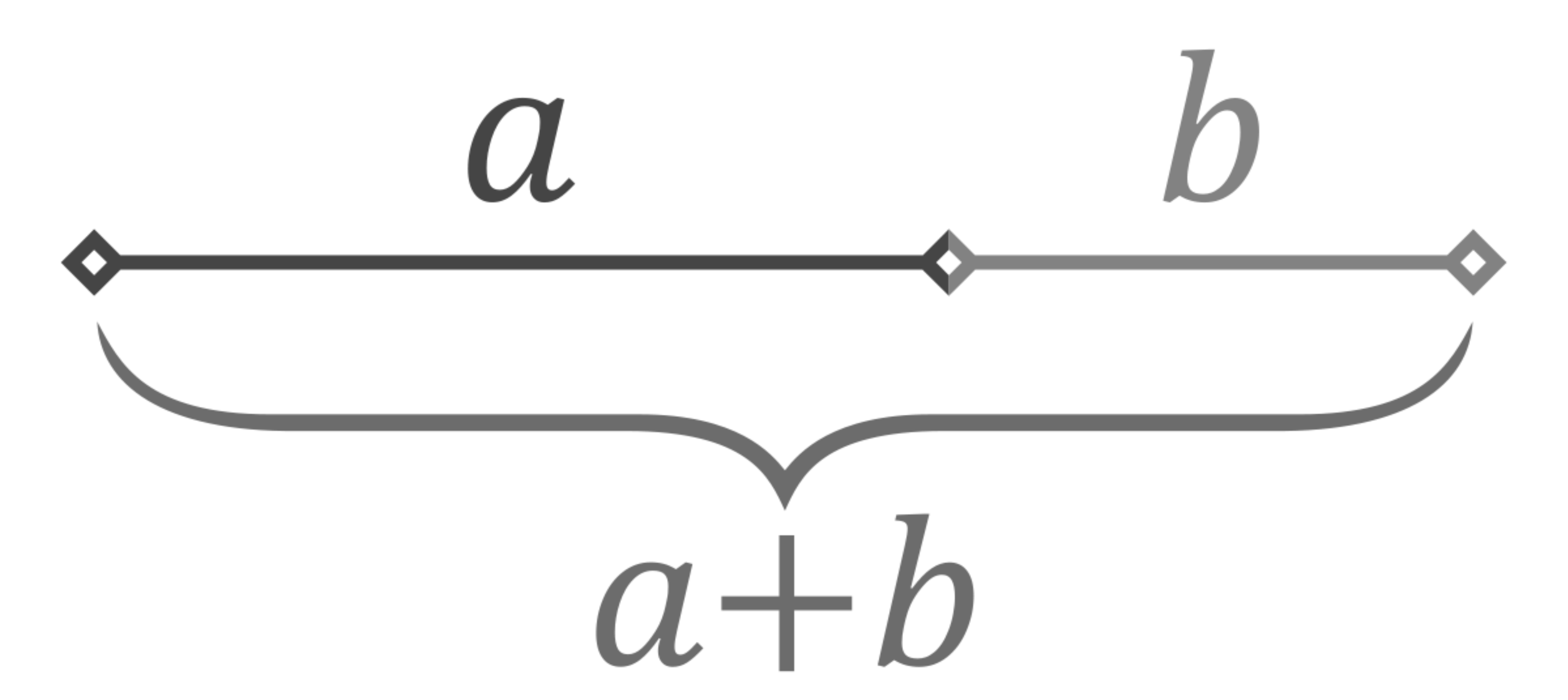# Golden Ratio Calculator

Created by Piotr Małek and Mateusz Mucha
Reviewed by Bogna Szyk and Jack Bowater
Last updated: Mar 11, 2022

The golden ratio calculator will calculate the shorter side, longer side and combined length of the two sides to compute the golden ratio. Before we can calculate the golden ratio it's important to answer the question "what is the golden ratio?". The following section will hope to provide you with an answer.

## What is golden ratioThe golden ratio, also known as the golden section or golden proportion, is obtained when two segment lengths have the same proportion as the proportion of their sum to the larger of the two lengths. The value of the golden ratio, which is the limit of the ratio of consecutive Fibonacci numbers, has a value of approximately 1.618.

The formula for the golden ratio is as follows. Let the larger of the two segments be a and the smaller be denoted as b The golden ratio is then (a+b)/a = a/b Any old ratio calculator will do this trick for you, but this golden ratio calculator deal with this issue specifically so you don't have to worry!

Here's a step by step method to solve the ratio by hand.

1. Find the longer segment and label it a
2. Find the shorter segment and label it b
3. Input the values into the formula.
4. Take the sum a and b and divide by a
5. Take a divided by b
6. If the proportion is in the golden ratio, it will equal approximately 1.618
7. Use the golden ratio calculator to check your result

The segment addition postulate can be used to find one of the segment lengths when 3 points are collinear, and two of the distances are known.

## Golden rectangle

The golden rectangle is a rectangle with a length of a+band width of a. This rectangle is often seen in art, as it has been said it's the most pleasing to the eye of all such rectangles. The golden rectangle calculator is a convenient way to find the golden rectangle instead of working it by hand.

The golden ratio is seen in many forms of architecture and in some patterns of nature, such as in the arrangement of leaves in some plants. The golden proportion is also seen in regular pentagons.

Piotr Małek and Mateusz MuchaLonger section (a)
ft
Shorter section (b)
ft
Whole (a+b)
ft
People also viewed…

### Body fat

Use the body fat calculator to estimate what percentage of your body weight comprises of body fat.

### Grams to cups

The grams to cups calculator converts between cups and grams. You can choose between 20 different popular kitchen ingredients or directly type in the product density.

### Improper fraction to mixed number

Use this improper fraction to mixed number calculator to convert quickly between these two fraction forms.

### Midpoint

Use the midpoint calculator to find out the midpoint of a line segment, which is the point that cuts the segment into two equal parts.